An Algebraic Integer of the form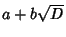whereis Squarefree forms a quadratic field and is denoted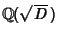. If, the field is called a Real Quadratic Field, and if, it is called an Imaginary Quadratic Field. The integers inare simply called the'' Integers. The integers inare called Gaussian Integers, and the integers in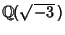are called Eisenstein Integers. The Algebraic Integers in an arbitrary quadratic field do not necessarily have unique factorizations. For example, the fieldsandare not uniquely factorable, since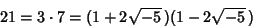(1)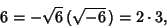(2)

although the above factors are all primes within these fields. All other quadratic fieldswith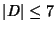are uniquely factorable.(3)(4)

and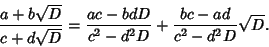(5)

The Integers in the real fieldare of the form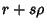, where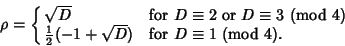(6)

There exist 22 quadratic fields in which there is a Euclidean Algorithm (Inkeri 1947).